Measure in a topological vector space

A term used to designate a measure given in a topological vector space when one wishes to stress those properties of the measure that are connected with the linear and topological structure of this space. A general problem encountered in the construction of a measure in a topological vector space is that of extending a pre-measure to a measure. Letbe a (real or complex) locally convex space and let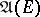be the algebra of its cylindrical sets (cf. Cylinder set). Suppose that a pre-measure is defined on. It is required to extend this pre-measure to a countably-additive measure defined on the-algebra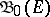— the smallest-algebra containing.is the smallest of all-algebras (weakly Borel, Borel, etc.) that are naturally connected with the topology of; for a large class of spacesthese-algebras coincide. In the particular, yet most important, case when the space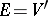, i.e. it is the dual of some locally convex space, endowed with the weak-* topology (so that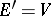), in order that a pre-measurein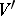admits an extension to a measure, it suffices that its characteristic functional (Fourier transform)be continuous in the so-called Sazonov topology on the space(i.e. in the topology generated by all continuous Hilbert semi-norms in) and in a number of cases — for example, ifis a Fréchet space — it is necessary that the characteristic functional be continuous in the original topology of. For instance, ifis a nuclear space, the Sazonov topology is identical with the original topology, and every pre-measure inwith a continuous characteristic functional extends to a measure. In the case of a pre-measure defined on a Hilbert spacethe sufficient condition for its extendability to a measure formulated above is also necessary. In addition to this general criterion for the extendability of pre-measures to measures, partial results of this type, applicable to specific classes of measures (or classes of spaces), are available. For example, a Gaussian pre-measure on, whereis a locally convex space (i.e. a pre-measure the restriction of which to any-algebra,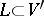,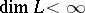, is a Gaussian distribution with correlation functional,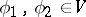) extends to a measure if there exists a convex neighbourhood of zero inwith-entropy, in the metric defined by the inner product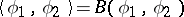, smaller than two.
For a sequence of (probability) measures in a dual spaceto converge weakly it suffices that the characteristic functionals of these measures converge pointwise (this condition is also necessary) and that they be equicontinuous at zero in the Sazonov topology in, and it is necessary that these functionals be equicontinuous in the original topology of. In the case of a Hilbert space, conditions necessary and sufficient for the weak compactness of a family of measures inare known. These are also expressible in terms of their characteristic functionals. The following aspects have been investigated (1982) only for Gaussian measures: the problem of the quasi-invariance of a measure in a topological vector space (see Quasi-invariant measure) with respect to some set of translations (the set of quasi-invariance) of this space (it is known that for a number of infinite-dimensional vector spaces the set of quasi-invariance of a non-zero measure does not necessarily coincide with the whole space); and criteria for the absolute continuity of one measure with respect to another. The study of measures in topological vector spaces is mainly connected with integrals over trajectories (cf. Integral over trajectories), and also with the theory of generalized random fields, and is to a high degree stimulated by the applications of these theories in physics and mechanics.